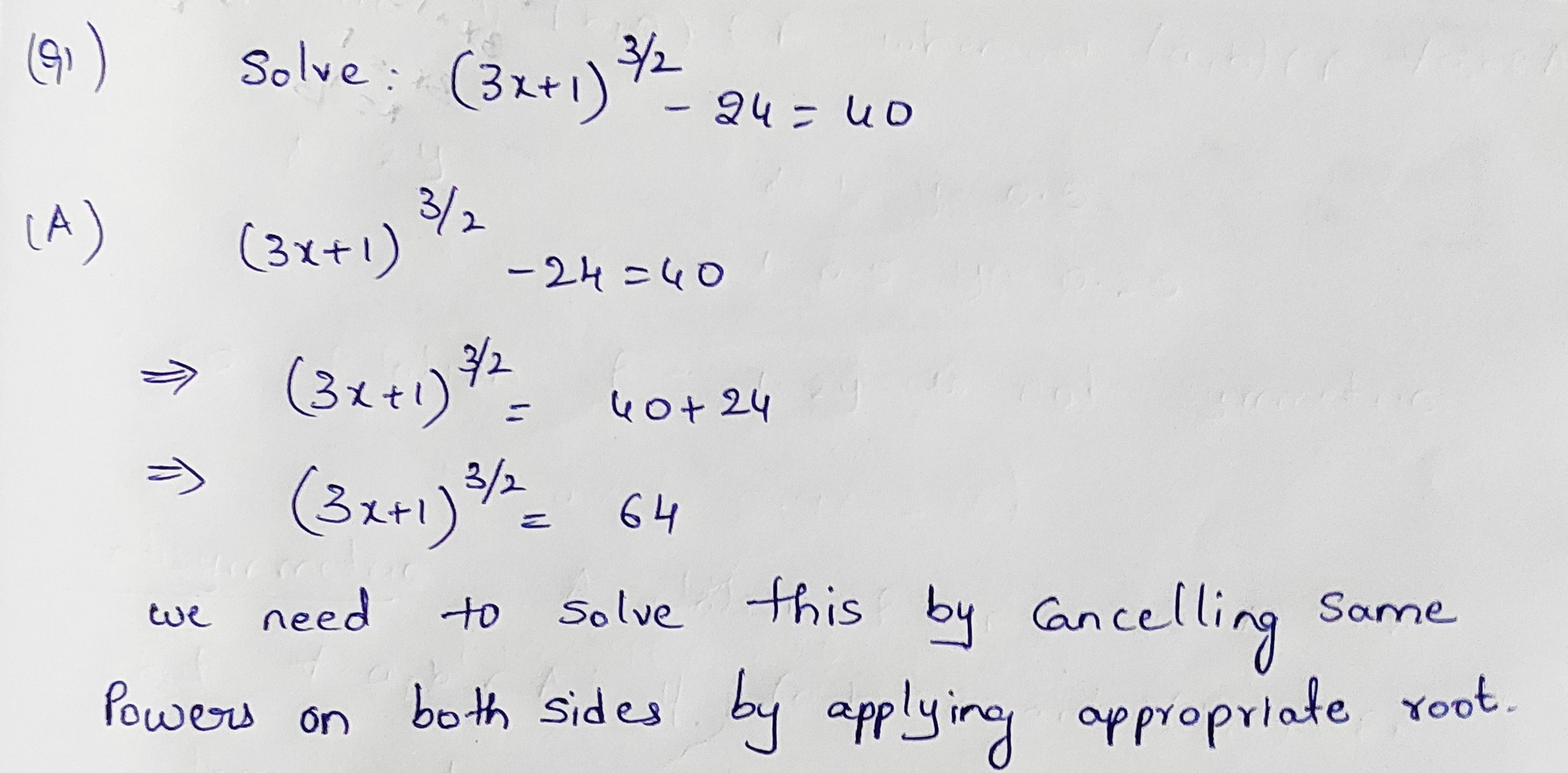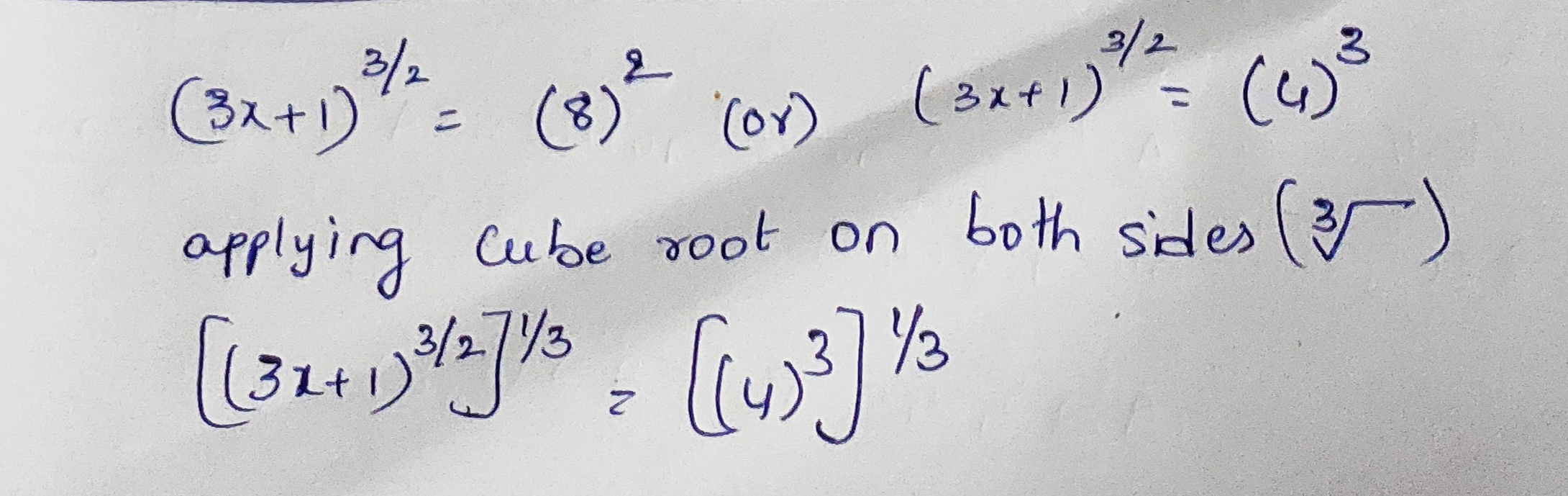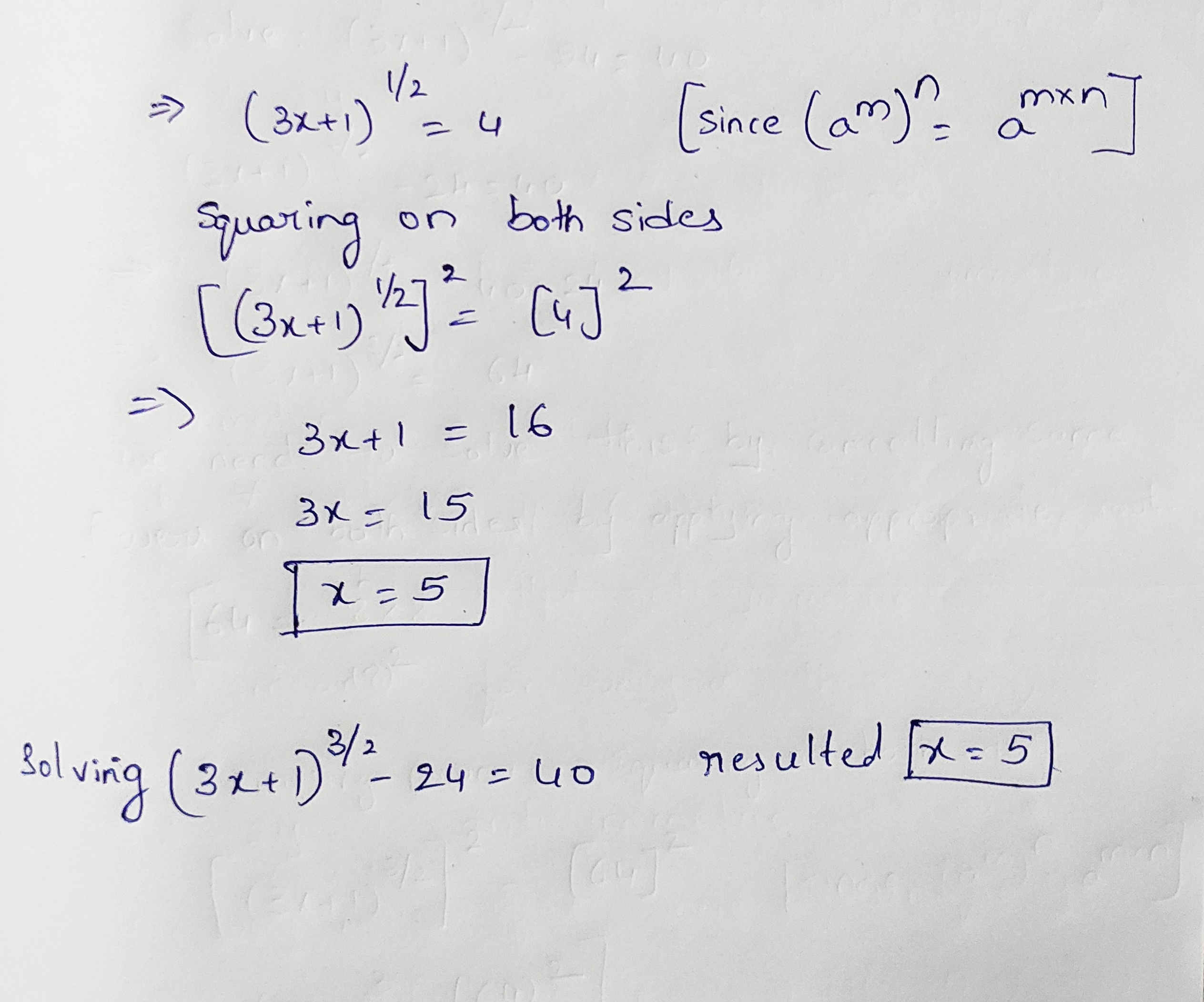In: Math

# Solve polynomial equation.

Solve the mathematical equation (3x+1)^(3/2) - 24 = 40.

## Solutions

##### Expert Solution

This question deals with the topic of Exponents and Powers.

If we do by elaborating the equation by powers, it results complicated expansion.

trick is to apply powers appropriately to equation as solved below.

We used the simple formula without complicating by going $${m}$$to expand the equation.

formula : $$(a^{m})^{n} = a^{m*n}$$Solving the equation using concept of exponents and powers.

upon Solving

X = 5.